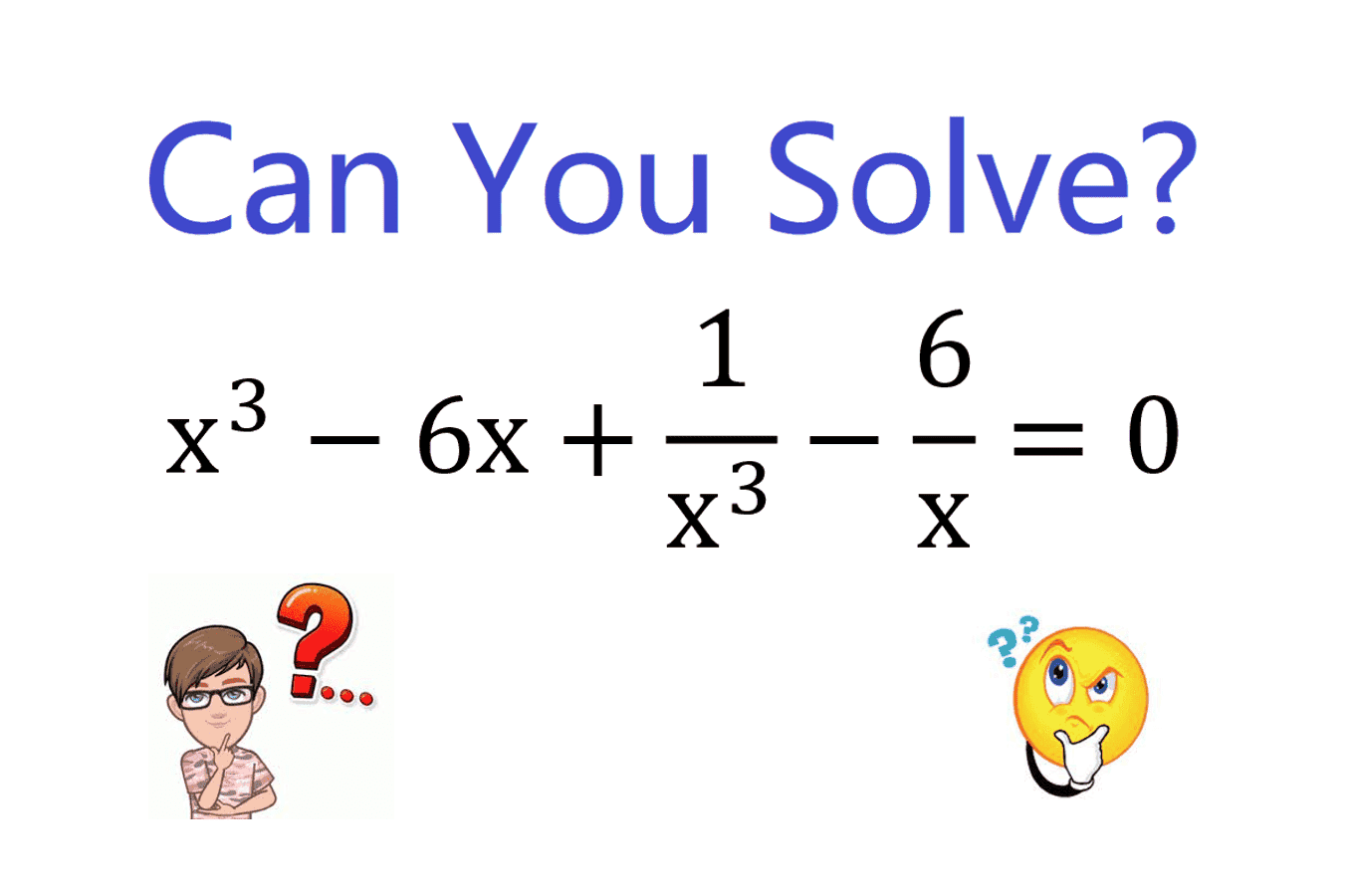# Can you solve this double cubic algebraic equation?

• MHB
• DrLiangMath

#### DrLiangMathThe key idea is to view $x+\frac{1}{x}$ as a whole. There are four real solutions: $x=\frac{3±\sqrt{5}}{2}$, $x=\frac{-3±\sqrt{5}}{2}$ (and two complex solutions: $x=±i$). Here is the explanation:

The key idea is to view $x+\frac{1}{x}$ as a whole. There are four real solutions: $x=\frac{3±\sqrt{5}}{2}$, $x=\frac{-3±\sqrt{5}}{2}$ (and two complex solutions: $x=±i$). Here is the explanation:

Nice idea!

Or you could just multiply both sides by $x^3$ and sub in $y = x^2$. The resulting cubic equation for y is easy to solve using the rational root theorem.

-Dan

•mathwonk
Nice idea!

Or you could just multiply both sides by $x^3$ and sub in $y = x^2$. The resulting cubic equation for y is easy to solve using the rational root theorem.

-Dan
Thank you very much for your feedback!

Derek